# Deep learning library roundup: keras vs tensorflow vs pytorch

I've been working on deep learning related projects since 2011. In the past 6 years, a lot has changed. One area of change is the seemingly endless introduction of new deep learning frameworks. It can be hard to keep up with the latest and greatest. I'm going to talk about some recent experiments I've been doing with three popular deep learning frameworks: Keras, Pytorch, and Tensorflow.

## The legacy of theano

I started my long descent into madness as a theano user, which provided automatic differentiation, gpu support, and a bunch of helper functions. GPU-support and autodifferentiation were the killer features, but theano itself had a very steep learning curve.

### Hardware: In 2012 I was using GTX 580s, which cost about \$300 and had 3gb of RAM. Now I'm using a Titan Xs with 12gb of ram at a cost of \$1,500. Better performance, but somehow not getting cheaper... 3gb is easy to fill up on a GPU. 12gb can handle a fairly large network though.

Several frameworks were built around theano. Pylearn2 was the one I favored. Lasagna and Blocks were two other examples.

There were other deep learning frameworks, maybe most notably Caffe, torch, and DL4J. I was never a big user of these because I really like python and really liked theano's model. DL4J is the go-to if you have to use java, and torch I will talk about shortly.

I did use caffe a couple times a few years back because they had some up-to-date layer type that I wanted to use. I found Caffe to be somewhat of a bear to work with, but your mileage may vary. A lot of people have switched from caffe to tensorflow though.

## The legacy of torch -> keras, tensorflow, pyTorch

When Keras came out, it was basically trying to take the torch api and put it into python. This turned out to be a really good idea.

Torch's main failing is it's written in lua. Of course, that's like saying Ruby On Rails' main failing is that it's written in ruby, which is simply untrue if you're a ruby fan. But my point is this: python has critical mass among data scientist types and torch's api in python brought a more elegant model than frameworks like pylearn2 while giving easy access to the rest of the python eco-system. Keras used theano as a backend for GPU and auto-differentiation support, following in the tradition of other python-based deep learning libraries.

Then tensorflow came out from google, and a lot of people were very excited about that because, well, it's google. Keras also introduced support for tensorflow as a backend. As far as I can tell, a lot of people are using tensorflow at this point.

Then a new python library based on torch's api (but in python) came out, called pyTorch. Keras and pyTorch come from the same philisophical idea, but they're a bit different in practice, as I'll show. Also, while keras is somewhat of a wrapper around theano and/or tensorflow, pyTorch rolled their own auto-differentiation and gpu support.

# Experiments and Code

Every once in a while, I go through a bunch of the deep learning frameworks and try them on some problems I have setup. I recently did this with tensorflow, keras, and pytorch.

## Experiment Setup

So I've got a dataset that is basically 5 years of stock market data. I like to use this dataset to test libraries because it's a difficult timeseries prediction problem, but there is signal. It's not a bad benchmark dataset, and if I ever get it working really well, I can start a hedge fund.

I'm going to build similar models in keras, tensorflow, and pytorch. These will basically be LSTMs with 2 output targets, a softmax output, and a real numbered vector output. It's setup as a transfer learning problem where a sequence vector is generated, and then a probability distribution and a set of real values are output and trained with separate cost functions.

The primary problem with this dataset is overfitting. That's been a consistent problem. If you don't use test data, you can train the model to literally "predict the stock market" with ~97% accuracy, but it's useless because it fails on test data.

Here's what your portfolio performance looks like when you overfit your model and then run a backtest against your training data. 100 on the y-axis is a starting portfolio value so ending up at 500 is a 500% return in a few months.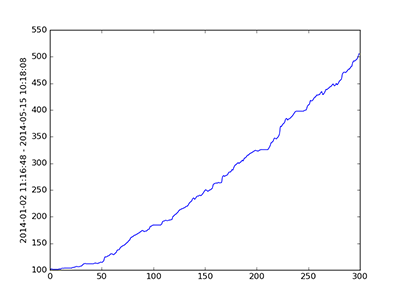If you could trade like this, the most logical conclusion would be that you're from the future. Theoretically, you could trade options for higher gains, but my main point is it's easy to overfit a model to this data and the resulting model is absolutely useless for prediction on heldout data.

So cross validation is pretty key here, and overfitting is a bad sign and something I'm very interested in combatting. I use transfer learning because you can basically use "the state of the market" to predict multiple things, and this seems to help training a bit.

``````The basic model is: input_data -> (lstm rolls up input into single vector) -> relu -> output
``````

This is very similar to a "sequence to sequence" model except it's "sequence to single label".

# Keras

This is the library I've been using the longest. Here's the code:

``````import keras
from keras.models import Sequential, Model, load_model
from keras.layers import Dense, Activation, Flatten, LSTM, SimpleRNN, Input, Merge, GRU
from keras.layers.noise import GaussianNoise
from keras.optimizers import Adam
from keras.callbacks import EarlyStopping
from keras.initializers import RandomUniform, TruncatedNormal, glorot_uniform
from keras.callbacks import Callback
from keras.utils import to_categorical

class PlotLossHistory(keras.callbacks.Callback):
"""
We use this to plot our losses during training. Keras provides
nice callback functionality.
"""

def on_train_begin(self, logs={}):
self.test_loss_softmax = []
self.test_loss_mse = []
self.train_loss_softmax = []
self.train_loss_mse = []

def on_epoch_end(self, batch, logs={}):
plt.clf()

fig = plt.figure()
ax1 = fig.add_subplot(111)

self.train_loss_softmax.append( logs['output_softmax_loss'] )
ax1.plot(self.train_loss_softmax)

self.test_loss_softmax.append( logs['val_output_softmax_loss'] )
ax1.plot(self.test_loss_softmax)

ax2 = ax1.twinx()

self.train_loss_mse.append( logs['output_predictions_loss'] )
ax2.plot(self.train_loss_mse)

self.test_loss_mse.append( logs['val_output_predictions_loss'] )
ax2.plot(self.test_loss_mse)
ax2.set_yscale('log')

#plt.legend(['TRAIN softmax', 'TRAIN mse', 'TEST softmax', 'TEST mse'], loc='upper right')

plt.savefig('output_losses.png')

# create entry point for model input
inputs = Input(shape=input_shape)

# setup model
# initializer = RandomUniform(-.001, .001)
lstm = LSTM(128)
timesteps_rolled_up = lstm(inputs)
relu = Dense(32, activation="relu")(timesteps_rolled_up)
output_prediction = Dense(num_outputs_linear, activation="linear", name="output_predictions")(relu)
output_softmax = Dense(num_outputs_softmax, activation="softmax", name="output_softmax")(relu)

model = Model(inputs=[inputs], outputs=[output_softmax, output_prediction])

# setup training and compile graph
opt = Adam(lr=0.0001, clipnorm=10.)
model.compile(loss={"output_softmax": "categorical_crossentropy", "output_predictions": "mse"},
optimizer=opt,
metrics=['accuracy',])

# one last fix to the data -- go from integer to 1-hot vectors suitable for softmax outputs
data_Y1 = to_categorical(np.int32(data_Y1), num_outputs_softmax)
test_Y1 = to_categorical(np.int32(test_Y1), num_outputs_softmax)
# if validation loss stops improving and/or starts overfitting too much, we'll stop training
early_stop = EarlyStopping('val_loss', .0001, patience=3, verbose=1, mode='auto')
# train your model, coffee break
model.fit(data_X, {"output_softmax": np.int32(data_Y1), "output_predictions": data_Y2},
epochs=epochs, batch_size=128,
validation_data=(test_X, {"output_softmax": np.int32(test_Y1), "output_predictions": test_Y2}),
shuffle=True, callbacks=[early_stop, PlotLossHistory()])

print 'done training'
# model.predict(data_X[:1]) # make a single prediction with your model
``````

When I train, I have it output training and testing errors. Here's what the chart looks like. This took 2-3 hours to train each model. Green lines are validation error.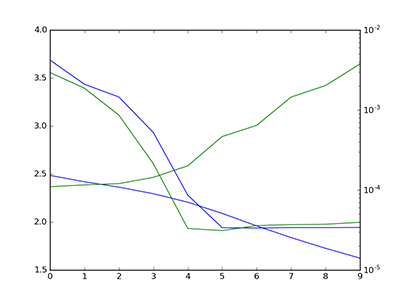Overfitting too quickly, reduce learning rate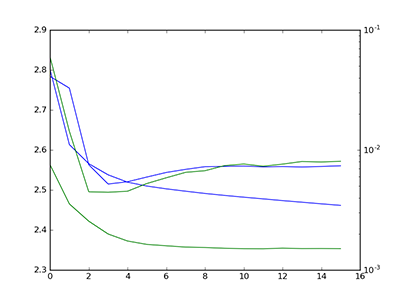Better, run backtest.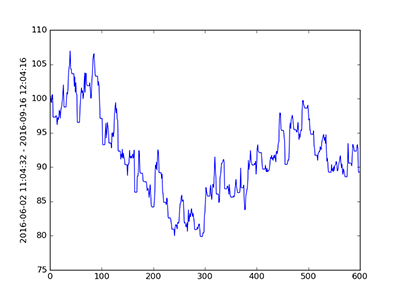Not so much.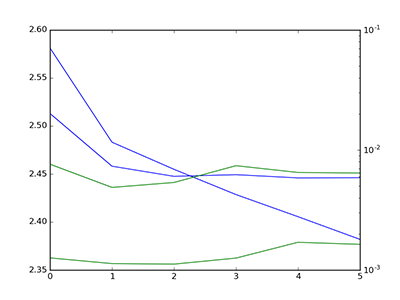Lower learning rate, mess with initialization parameters.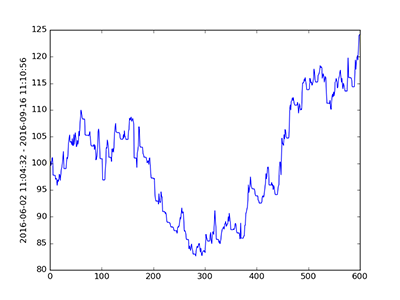We end on a high note but those drawdowns are brutal.

Overall: Performance here (on the dataset, the library performs fine) isn't fantastic. I should probably be using keras functional API to get more customizability.

It looks like I didn't train for very long, but each epoch represents a full scan through the dataset dims: (120000, 200, 192), and the other experiments count an epoch after seeing a smaller number of samples. So even though it looks like it's converging/diverging after a small number of epochs, it actually takes a while.

Keras can be very easy to get started with (and defaults can work quite well), but it also provides mechanisms to customize your model more deeply.

# Tensorflow

My general impression of tensorflow is its overall model is "less elegant". It's easy enough to setup, and I used it here specifically because they had a certain type of LSTM unit I wanted to use. Tensorflow really does seem to be the most "feature complete" deep learning library.

here's the code:

``````import tensorflow as tf
sess = tf.Session()

class TFdataset:
"""
this will hold our data and labels and handle shuffling
"""

def __init__(self, data_X, data_Y1, data_Y2, batch_size):
self.data_X = data_X
self.data_Y1 = to_categorical(data_Y1, np.max(data_Y1)+1) # softmax
self.data_Y2 = data_Y2 # mse
self.batch_size = batch_size
pass

def iter(self):
batch_idx = 0
while True:
# shuffle data
idxs = np.arange(0, len(self.data_X))
np.random.shuffle(idxs)
shuf_features = self.data_X[idxs]
shuf_Y1 = self.data_Y1[idxs]
shuf_Y2 = self.data_Y2[idxs]

for batch_idx in range(0, len(self.data_X), self.batch_size):
data_batch = shuf_features[batch_idx: batch_idx+self.batch_size]
data_batch = data_batch.astype('float32')
Y1_batch = shuf_Y1[batch_idx: batch_idx+self.batch_size]
Y2_batch = shuf_Y2[batch_idx: batch_idx+self.batch_size]

yield data_batch, Y1_batch, Y2_batch

# params =========================
BATCH_SIZE = 128
BATCH_SIZE_TEST = 1024
LSTM_SIZE = 128
LEARNING_RATE = .001
training_iters = 4000
display_step=20
TIMESTEPS = 200
DROPOUT = 1. # 1. is no dropout
#=================================

# setup your data iterators
dset_train = TFdataset(data_X, data_Y1, data_Y2, BATCH_SIZE)
dset_test = TFdataset(test_X, test_Y1, test_Y2, BATCH_SIZE_TEST)

data_iterator = dset_train.iter()

# variables we'll use shortly
n_steps = TIMESTEPS
n_input = num_inputs
n_outputs_sm = num_outputs_softmax
n_outputs_mse = num_outputs_linear

# setup input X and output Y graph variables
x = tf.placeholder('float', [None, n_steps, n_input])
y = tf.placeholder('float', [None, n_outputs_sm])

# init information for model weights
weights = {
'out': tf.Variable(tf.random_uniform([LSTM_SIZE, n_outputs_sm]))
}
biases = {
'out': tf.Variable(tf.random_uniform([n_outputs_sm]))
}

# Feed forward function to get the RNN output. We're using a fancy type of LSTM cell.
def TFEncoderRNN(inp, weights, biases):
# current_input_shape: (batch_size, n_steps, n_input
# required shape: 'n_steps' tensors list of shape (batch_size, n_input)
inp = tf.unstack(inp, n_steps, 1)
lstm_cell = tf.contrib.rnn.LayerNormBasicLSTMCell(LSTM_SIZE, dropout_keep_prob=DROPOUT)
outputs, states = tf.contrib.rnn.static_rnn(lstm_cell, inp, dtype=tf.float32)
return tf.matmul(outputs[-1], weights['out']) + biases['out']

# we'll be able to call this to get our model output
pred = TFEncoderRNN(x, weights, biases)
# define loss
cost = tf.reduce_mean(tf.nn.softmax_cross_entropy_with_logits(logits=pred, labels=y))

# learning rate decay
global_step = tf.Variable(0, trainable=False)
lr_scheduler = tf.train.exponential_decay(LEARNING_RATE, global_step,
training_iters, 0.96, staircase=True)
# define optimizer
optimizer = tf.train.AdamOptimizer(learning_rate=lr_scheduler).minimize(cost)

# define evaluation params
correct_pred = tf.equal(tf.argmax(pred,1), tf.argmax(y,1))
accuracy = tf.reduce_mean(tf.cast(correct_pred, tf.float32))

init = tf.global_variables_initializer()

# train model
config = tf.ConfigProto(allow_soft_placement=True, log_device_placement=True)
config.gpu_options.allow_growth = True

sess = tf.Session(config=config)

sess.run(init)
step = 1

print colored('start training', 'blue')
softmax_loss_history = []
training_accuracy = []
softmax_loss_history_test = []
test_accuracy_history = []

# model training loop
data_iterator = dset_train.iter()
test_iterator = dset_test.iter()
while step < training_iters:
batch_x, batch_sm, batch_mse = data_iterator.next()

sess.run(optimizer, feed_dict={x: batch_x, y: batch_sm})

if step % display_step == 0:

data_iterator = dset_train.iter()
test_iterator = dset_test.iter()

# get training stats
acc = sess.run(accuracy, feed_dict={x: batch_x, y: batch_sm})
loss = sess.run(cost, feed_dict={x: batch_x, y: batch_sm})
softmax_loss_history.append(loss)
training_accuracy.append(acc)

# get test data stats
test_X, test_softmax, test_mse = test_iterator.next()

acc_test = sess.run(accuracy, feed_dict={x: test_X, y: test_softmax})
loss_test = sess.run(cost, feed_dict={x: test_X, y: test_softmax})

softmax_loss_history_test.append(loss_test)
test_accuracy_history.append(acc_test)

print 'Iter %i, Minibatch Loss= %.6f, Training Accuracy= %.5f' % (step, loss, acc)
pytorch_plot_losses(softmax_loss_history=softmax_loss_history,
mse_loss_history=training_accuracy,
test_losses_softmax=softmax_loss_history_test,
test_losses_mse=test_accuracy_history)
step += 1

print "Optimization finished!"
``````

I'm not sure if I was just doing something wrong or what, but tensorflow seems to be slow as hell (as compared to other GPU-enabled deep learning frameworks). Each model took 4-12 hours to train (and it doesn't use multiple outputs so it actually has less computation to do than keras and pytorch in my examples.)

Here's the training plots: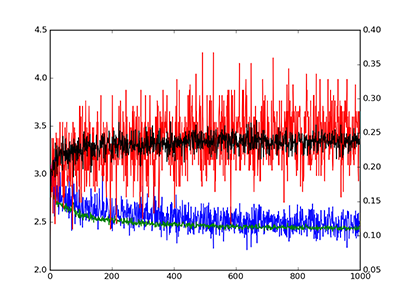50% dropout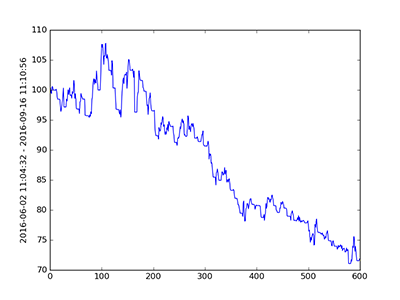oops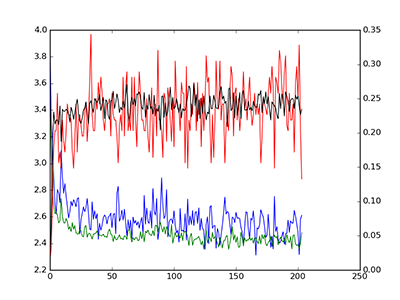20% dropout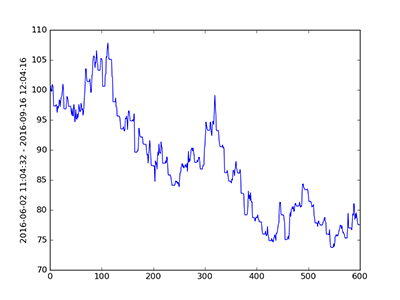also bad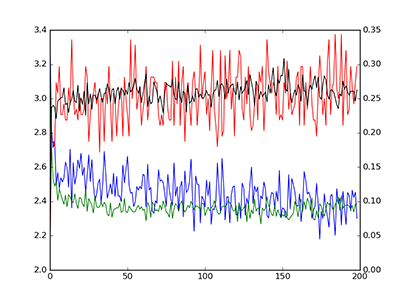no dropout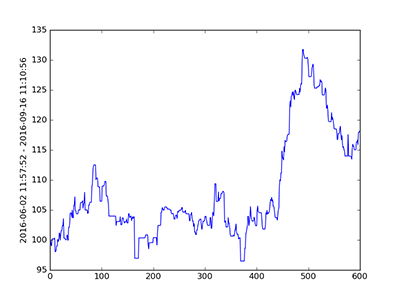(no dropout) promising...

Overall: I've found tensorflow to be really cool, and the performance seems promising so I'll be developing it out further and investigating different architecture combinations. It seems to be the best solution to my overfitting problem so far, so I want to see if I can at least pull out a bit more raw performance.

Also, I didn't set this up as a transfer learning problem here. That can mean two things: adding transfer learning will help my performance, or transfer learning is hurting my performance in the other library experiments. Must run more experiments...

On the downside, it does seem awfully slow, making it harder for me to experiment.

# pyTorch

Pytorch is pretty cool. It's similar to keras, but keras has... more batteries included? For instance, I don't have to write my own training loop in keras. But you arguably gain more control if you write it yourself. Honestly, it's not a make or break difference.

One thing I like about pytorch is the whole concept of taking a data tensor and going "data_x.cuda()" to put it on GPU and "data_x.cpu()" to take it off GPU. You can see the results immediately through a "watch nvidia-smi" process. Pytorch has a "high level but low level" feel to it in the sense of the overall model is elegant (based on torch), but it provides certain low level hardware access that was much more of a pain to work with in eg theano.

The fact that this is the longest code example by far should not be taken as an indictment of how much code you have to write to use pyTorch. Although, in comparison to keras, it's rather verbose. Overall, I could see myself using this library more and I will probably try tweaking this code further as I'm sure there are some things I'm doing incorrectly.

here's the code:

``````from torch.autograd import Variable
import torch.optim as optim
import torch.nn.functional as torch_func
import torch

def pytorch_plot_losses(softmax_loss_history=None, mse_loss_history=None,
test_losses_softmax=None, test_losses_mse=None):
plt.clf()
fig = plt.figure()
ax1 = fig.add_subplot(111)
if softmax_loss_history:
ax1.plot(softmax_loss_history, color="blue")
if test_losses_softmax:
ax1.plot(test_losses_softmax, color="green")
ax2 = ax1.twinx()
if mse_loss_history:
ax2.plot(mse_loss_history, color="red")
if test_losses_mse:
ax2.plot(test_losses_mse, color="black")
#ax2.set_yscale('log')
plt.savefig('output_losses.png')

class EncoderRNN(nn.Module):
"""
pytorch class to encode a series of timesteps into a single vector
"""
def __init__(self, num_inputs, hidden_units, num_outputs, batch_size):
super(EncoderRNN, self).__init__()
self.batch_size = batch_size
self.num_inputs = num_inputs
self.hidden_units = hidden_units
self.lstm = nn.LSTM(num_inputs, hidden_units, num_layers=1, batch_first=True).cuda()
self.relu = nn.ReLU().cuda()
self.fc_out = nn.Linear(hidden_units, num_outputs).cuda()
n = hidden_units * num_inputs
self.lstm.weight_hh_l0.data.normal_(0, np.sqrt(2. / n))
self.lstm.weight_ih_l0.data.normal_(0, np.sqrt(2. / n))
self.fc_out.weight.data.normal_(0, np.sqrt(2. / n))

def forward(self, input, hidden):
output, hiddens = self.lstm(input, hidden)
ret_output = self.relu(self.fc_out(output[:,-1,:]))
return ret_output

def initHidden(self, batch_size):
result = Variable(torch.zeros(1, batch_size, self.hidden_units))
return result.cuda()

class DecoderRNN(nn.Module):
"""
Given an encoded hidden state, can decode into real values or a softmax
"""

def __init__(self, input_size, hidden_size, output_size, output_type=None):

super(DecoderRNN, self).__init__()

self.hidden_size = hidden_size
self.output_size = output_size
self.output_type = output_type

n = hidden_size * input_size
self.fc1 = nn.Linear(input_size, hidden_size).cuda()
self.relu = nn.ReLU().cuda()
self.fc_output = nn.Linear(hidden_size, output_size).cuda()

self.fc1.weight.data.normal_(0, np.sqrt(2. / n))
self.fc_output.weight.data.normal_(0, np.sqrt(2. / n))

if output_type=="softmax":
self.softmax = nn.LogSoftmax().cuda()
elif output_type=="linear":
self.softmax = None
else:
raise Exception('Initializing decoder RNN without supplying "output_type"')

def forward(self, input):
relu_out = self.relu( self.fc1( input ) )
output = self.fc_output( relu_out )
if self.output_type=='softmax':
# apply softmax to output to get probablity distribution,
# otherwise just output raw output values
output = self.softmax(output)
else:
output = torch_func.tanh(output)
return output

class PytorchStockDataset(tdata.Dataset):
"""
Pytorch wants you to define a fancy dataset wrapper class
"""

def __init__(self, data_X, Y_softmax, Y_mse, inflate_y=False):
assert len(data_X) == len(Y_softmax) == len(Y_mse)
if inflate_y:
self.data_Y_softmax = to_categorical(np.float32(Y_softmax.squeeze()), np.max(Y_softmax)+1)
self.data_X = data_X
self.data_Y_mse = Y_mse
else:
self.data_Y_softmax = torch.from_numpy(Y_softmax)
self.data_X = torch.from_numpy(data_X)
self.data_Y_mse = torch.from_numpy(Y_mse)

def __getitem__(self, index):
x = self.data_X[index]
y_mse = self.data_Y_mse[index]
y_softmax = self.data_Y_softmax[index]
return x, y_mse, y_softmax

def __len__(self):
return len(self.data_X)

# params =======================
BATCH_SIZE = 128
BATCH_SIZE_TEST = 1024
LSTM_SIZE = 128
ENCODER_OUTPUT_SIZE = 1024
RELU_SIZE = 512
lr_encoder = .0001
lr_decoder_softmax = .0005
lr_decoder_mse = .0001
# =============================

# setup data for pytorch
dset_train = PytorchStockDataset(data_X, Y_softmax=data_Y1, Y_mse=data_Y2)
dset_test = PytorchStockDataset(test_X, Y_softmax=test_Y1, Y_mse=test_Y2)

data_sampler_train = tdata.DataLoader(dset_train, batch_size=BATCH_SIZE, shuffle=True, drop_last=True)
data_sampler_test = tdata.DataLoader(dset_test, batch_size=BATCH_SIZE_TEST, shuffle=False, drop_last=True)

# init your encoder and two decoders
encoder = EncoderRNN(num_inputs, LSTM_SIZE, ENCODER_OUTPUT_SIZE, batch_size=BATCH_SIZE)
decoder_softmax = DecoderRNN(ENCODER_OUTPUT_SIZE, RELU_SIZE, num_outputs_softmax, output_type='softmax')
decoder_mse = DecoderRNN(ENCODER_OUTPUT_SIZE, RELU_SIZE, num_outputs_linear, output_type='linear')

# create your optimizers
optimizer_enc = torch.optim.Adam(encoder.parameters(), lr=lr_encoder)
optimizer_sm_dec = torch.optim.Adam(decoder_softmax.parameters(), lr=lr_decoder_softmax)
optimizer_mse_dec = torch.optim.Adam(decoder_mse.parameters(), lr=lr_decoder_mse)

# run training for N epochs
batches_per_epoch = 100
epoch_losses_softmax = []
epoch_losses_mse = []
test_losses_softmax = []
test_losses_mse = []

best_iteration = 0
best_validation_loss = 999.
best_encoder_params = None
best_decoder_params = None
best_decoder_paramse_mse = None

for i in range(250):

data_iterator = iter(data_sampler_train)
test_iterator = iter(data_sampler_test)

print 'EPOCH: %d' % i

# run each epoch for N training batches
iter_losses_mse = []
iter_losses_softmax = []

for iteration in range(batches_per_epoch):
batch_X, batch_mse, batch_softmax = data_iterator.next()

hx = encoder.initHidden(BATCH_SIZE)
cx = encoder.initHidden(BATCH_SIZE)

# clear our cached gradient
optimizer_enc.zero_grad()
optimizer_mse_dec.zero_grad()
optimizer_sm_dec.zero_grad()

# encode our sequence
var_X = Variable(batch_X, requires_grad=True).cuda()
encoder_output = encoder(var_X, (hx, cx))

# decode our sequence
decoded_mse = decoder_mse(encoder_output)
decoded_softmax = decoder_softmax(encoder_output)

# compute loss from output and labels
criterion_softmax = nn.CrossEntropyLoss()
var_y_sm = Variable(batch_softmax, requires_grad=False)[:,0].long().cuda()
loss_softmax = criterion_softmax(decoded_softmax, var_y_sm)

# do the same for the second target w/ different cost function
criterion_mse = nn.MSELoss()
var_y_mse = Variable(batch_mse, requires_grad=False).cuda()
loss_mse = criterion_mse(decoded_mse, var_y_mse)

# add those losses to our history for plotting purposes
iter_losses_mse.append(loss_mse.data.cpu().numpy())
iter_losses_softmax.append(loss_softmax.data.cpu().numpy())

# backprop losses
loss_mse.backward(retain_variables=True)
loss_softmax.backward(retain_variables=True)

# update weights from computed gradient
optimizer_mse_dec.step()
optimizer_sm_dec.step()
optimizer_enc.step()

#####
# run validation data
#####
test_X, test_mse, test_softmax = test_iterator.next()

hx = encoder.initHidden(BATCH_SIZE_TEST)
cx = encoder.initHidden(BATCH_SIZE_TEST)

# encode our sequence
test_var = Variable(test_X, requires_grad=False).cuda()
encoder_test_output = encoder(test_var, (hx, cx))

# decode our sequence
decoded_test_mse = decoder_mse(encoder_test_output)
decoded_test_softmax = decoder_softmax(encoder_test_output)

# compute loss from output and labels
criterion_softmax = nn.CrossEntropyLoss()
var_y_sm_test = Variable(test_softmax, requires_grad=False)[:,0].long().cuda()
loss_softmax = criterion_softmax(decoded_test_softmax, var_y_sm_test)

# do the same for the second target w/ different cost function
criterion_mse = nn.MSELoss()
var_y_mse_test = Variable(test_mse, requires_grad=False).cuda()
loss_mse = criterion_mse(decoded_test_mse, var_y_mse_test)

test_loss_mse = loss_mse.data.cpu().numpy()
test_loss_softmax = loss_softmax.data.cpu().numpy()

test_losses_mse.append(test_loss_mse)
test_losses_softmax.append(test_loss_softmax)

if test_loss_softmax < best_validation_loss:
print colored('NEW BEST DETECTED', 'green')
best_iteration = i
best_validation_loss = test_loss_softmax
# save state dicts containing weights
best_encoder_params = encoder.state_dict()
best_decoder_params = decoder_softmax.state_dict()
best_decoder_params_mse = decoder_mse.state_dict()
# clone params in state dicts so they don't get overwritten
for key in best_encoder_params.keys():
best_encoder_params[key] = best_encoder_params[key].clone()
for key in best_decoder_params.keys():
best_decoder_params[key] = best_decoder_params[key].clone()
for key in best_decoder_params_mse.keys():
best_decoder_params_mse[key] = best_decoder_params_mse[key].clone()

times_since_last_best = i - best_iteration
if times_since_last_best > 30:
# early stopping
break

'''
this was experimental code where i was trying to help overfitting by
(after a certain number of iterations with no validation loss improvement)
reload our best saved validation score and add noise to some of the weights,
reduce the learning rate, and keep optimizing. The thinking here is maybe it
can get bumped into a better location. This kind of helped? But it's very
much unnecessary and I'm leaving it in just in case anyone is interested.
'''
dropout_factor = .2
print colored('WE SHOULD REJIGGER THE WEIGHTS, VALIDATION HAS STOPPED IMPROVING', 'red')
best_iteration = i
keys = best_encoder_params.keys()
for key in keys:
dat = best_encoder_params[key]
best_encoder_params[key] = dat #+ torch.from_numpy(np.random.uniform(-.0000001,.0000001,dat.size())*np.random.binomial(1.,dropout_factor,dat.size())).cuda().float()
encoder.load_state_dict(best_encoder_params)

keys = best_decoder_params.keys()
for key in keys:
dat = best_decoder_params[key]
best_decoder_params[key] = dat #+ torch.from_numpy(np.random.uniform(-.0000001,.0000001,dat.size())*np.random.binomial(1.,dropout_factor,dat.size())).cuda().float()
decoder_softmax.load_state_dict(best_decoder_params)

keys = best_decoder_params_mse.keys()
for key in keys:
dat = best_decoder_params_mse[key]
best_decoder_params_mse[key] = dat #+ torch.from_numpy(np.random.uniform(-.0000001,.0000001,dat.size())*np.random.binomial(1.,dropout_factor,dat.size())).cuda().float()
decoder_mse.load_state_dict(best_decoder_params_mse)
#[x.size() for x in encoder.parameters()]
#import pdb; pdb.set_trace()
#noise = np.random.random()(params.std()*3)

# when we revert back to a spot near our original place,
# halve the learning rate also
if lr_encoder > .00005:
lr_encoder = lr_encoder * .8
lr_decoder_softmax = lr_decoder_softmax * .8
lr_decoder_mse = lr_decoder_mse * .8

optimizer_enc = torch.optim.Adam(encoder.parameters(), lr=lr_encoder)
optimizer_sm_dec = torch.optim.Adam(decoder_softmax.parameters(), lr=lr_decoder_softmax)
optimizer_mse_dec = torch.optim.Adam(decoder_mse.parameters(), lr=lr_decoder_mse)

####

# report losses (hopefully they're decreasing)
print 'MSE loss: %.8f' % np.mean(iter_losses_mse)
print 'SMX loss: %.8f' % np.mean(iter_losses_softmax)
epoch_losses_mse.append( np.mean(iter_losses_mse) )
epoch_losses_softmax.append( np.mean(iter_losses_softmax) )
pytorch_plot_losses( epoch_losses_softmax, epoch_losses_mse, test_losses_softmax, test_losses_mse)

# revert weights to "best weights"
print colored('*** reverting weights back to iteration %i, which was our best' % best_iteration, 'yellow')
encoder.load_state_dict(best_encoder_params)
decoder_softmax.load_state_dict(best_decoder_params)
decoder_mse.load_state_dict(best_decoder_params_mse)

print '========== optimization finished! ============'
``````

here's the training plots: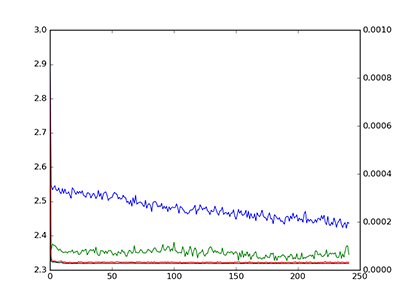initial loss curves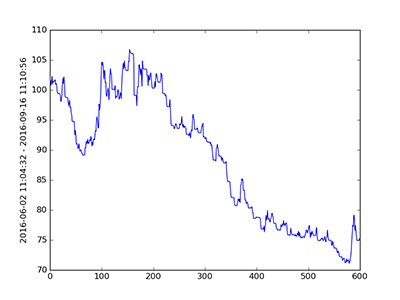not so good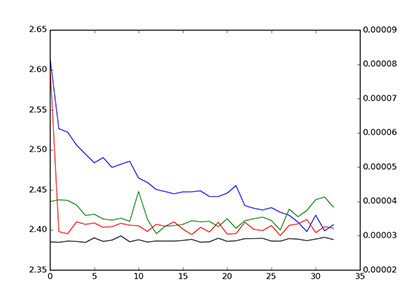after tweaking a bunch of parameters, especially random initialization settings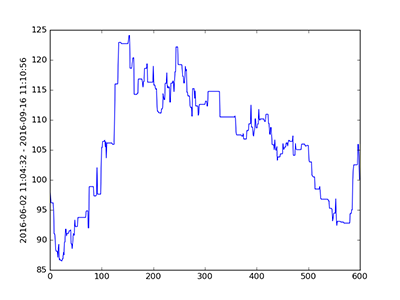a promising result

# closing thoughts

Overall, tensorflow, pytorch, and keras are really good libraries. They take a lot of things that used to be hard and make them easy. They make it possible to experiment with different concepts in deep learning very easily. They were written by people that really understand deep learning.

And these code examples are hardly comprehensive. A) I'm probably doing something suboptimal somewhere B) I haven't run a good hyperparameter search and C) I've focused on only LSTMs here where there are a lot of other configurations I could have used.

And I used Adam as an optimizer on all of these -- how do I know RMSProp or gradient descent with momentum wouldn't have worked better? How much have I played with learning rate schedules? Or injecting noise/adding dropout in different areas of the network? Or weight intialization schemes? How do I know TWEAK X wouldn't work better? The answer is, "I don't until I try it."

And that's what takes all the time in machine learning -- you're taking data and you're trying a bunch of different model architectures to see what works and what doesn't. The other piece that takes "all the time" is building/maintaining your data pipeline. That's the state of the art today.

So, which library should you use? I would say, "Start with Keras." If you've tried keras and want something different, try pytorch. If you want some new cutting edge feature and don't want to implement it yourself with pytorch or keras, try tensorflow.

PS - If you happen to be part of an engineering team that's looking to ramp-up their deep learning related activities and add predictive features to their products, you should hire me to help.